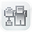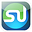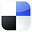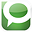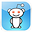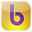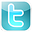What  Customers Are Saying  About  the Excel Statistical Master "We just started building statistical excel spreadsheets for our direct mail and online marketing campaigns, I purchased Excel Statistical Master to help fill in some of the blanks. Little did I know, this book has everything I could ever want to know about business statistics. Easy to follow and written so even a child could understand some of the most complex statistical theories. Thanks Mark!" Brandon Congleton Marketing Director www.worldprinting.com "After years of searching for a simplified statistics book, I found the Excel Statistical Master. Unlike the indecipherable jargon in the countless books I have wasted money on, the language in this book is plain and easy to understand. This is the best \$40 I have ever spent. " Mahdi Raghfar "I really like the Excel Statistical Master. It is incredibly useful. The explanations and videos in the manual are excellent. It has really made my work with statistics a LOT easier. I'm really glad that I came across the manual. If you're a student of business statistics, this e-manual is worth WAY more it's priced. I will use your manual as a reference for my MBA course this summer." Dr. Yan Qin Co-Director Nankai-Grossman Center for Health Economics and Medical Insurance
Excel
STATISTICAL
Master

Simple t Test Definition

Clear and Complete

# t Test Definition - Simplified

The t test is a statistics test generally used to determine whether the means of two populations are different. In the t test, a t value is calculated based upon the difference in the means and variances of samples drawn from each of the two populations. This t value is then compared to a critical t value, which is based upon your required level of certainty (perhaps you need to be at least 95% certain of the outcome) and the degrees of freedom present in the test. If the t value is greater than the critical t value, you can state that the 2 means are different, within your required degree of certainty.

The t value is calculated as follows:

t value = (Difference between the group means) / (Variability of the groups)

To sum up the above calculation; if the groups have a high degree of variance (noise), it is harder to tell whether there really is a difference in the means because the noise make both sample groups appear to blend together.

The higher amount of variance that exists in the groups, the higher will be the denominator of the above fraction. The higher the denominator, the lower will be the t value. The lower the t value, the less likely it is that the two means are different.

The means are considered different if the t value is greater than the critical t value. It is the t value comparison with the critical t value that determines whether there really is a difference between the means.

The t value's numerator (Difference between group means) and the denominator (variability of the groups) are calculated in difference ways for the different types of t tests.

The t test has a number of variations but the most common t test is used to determine whether the means of two normally-distributed populations are equal. This type of t test is referred to as a Student's t test, or sometimes just the student t test. Drawing samples from different populations in this fashion is referred to as an unpaired t test.

A one-sample t test uses one sample drawn from a single normally-distributed population to test whether the population's mean is equal to a specified value. In other words, the one-sample t test is used to test the Null Hypothesis that the population's mean is equal to that specified value. All other types of t tests are variations of the two-sample t test.

A paired t test or paired difference t test is use to determine whether "before" and "after" measurements taken of a group of objects are the same. The Null Hypothesis states that there is no difference between "before" and "after." Specifically, the Null Hypothesis states that "after" values minus "before" values equal 0.

The Student's t test works well if sample sizes are equal even when the populations being compared have unequal variances. A similar t test called the Welch's t test works fine for unequal sample sizes taken from populations with unequal variances.

The two populations from which samples are drawn must be determined to be normally distributed before the t Test can be applied. When a t Test is applied to populations that are not normally distributed, the calculated result is likely to be totally wrong.

The t test (specifically, the Student's t test) is equivalent to a one-way ANOVA test when it is being applied to test the equality of means of two normally distributed populations.

A one-sample t test uses one sample drawn from a single population to test whether the population's mean is equal to a specified value. In other words, the one-sample t test is used to test the Null Hypothesis that the population's mean is equal to that specified value.

A two-sample t test is used to test whether two separate normally-distributed populations have the same mean. The Student's t test works well if sample sizes are equal but the populations have different variances. Welch's t test works well for unequal sample sizes drawn from populations of unequal variance.

If You Like This, Then Share It...